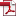# Software

Here follows a non-exaustive list of software I have produced. First I consider two "bigger" pieces of code and then a list of smaller educational ones, e.g. used for teaching.

## abc-sde

abc-sde a MATLAB toolbox for approximate Bayesian computation (ABC) in stochastic differential equation models.

It performs approximate Bayesian computation for stochastic models having latent dynamics defined by stochastic differential equations (SDEs). Both one- and multi-dimensional SDE systems are supported and partially observed systems are easily accommodated. Variance components for the "measurement error" affecting the data/observations can be estimated. A 50-pages Reference Manual is provided with two case-studies implemented and discussed. The methodology is based on the research article available here which also provide an additional example.
abc-sde has been partially supported by the Faculty of Science at Lund University during years 2012-2013.

Download abc-sdeReference manualA posterResearch paper 2013

## sde toolbox

Warning: implemented inferential methods are rather outdated and the SDE Toolbox development is discontinued.
SDE Toolbox - Simulation and estimation of stochastic differential equations with MATLAB.
This MATLAB package allows to simulate sample paths of a user defined Itô or Stratonovich SDE, estimate parameters and obtain descriptive statistics of the underlying stochastic process; users can also simulate an SDE chosen from a library of template models.

## Supporting code for my publications

Code associated to some of my papers are linked in my publications page. These can also be found on GitHub.

## pseudo-marginal MCMC for exact Bayesian inference

A MATLAB example of particle marginal MCMC algorithm to perform exact Bayesian inference for the parameters of a nonlinear state-space model. Available in my GitHub repository https://github.com/umbertopicchini/pseudomarginalMCMC.

## approximate Bayesian computation for g-and-k distributions

A MATLAB example of approximate Bayesian computation (ABC) MCMC algorithm to estimate parameters of a g-and-k distribution, at https://github.com/umbertopicchini/abc_g-and-k. See also my slides at http://goo.gl/ypAOjs.

## Fitting a stochastic Ricker model using the R pomp package

Several examples using the pomp package to implement several types of inference methods for the parameters of a stochastic Ricker model. Examples include: synthetic likelihoods; particle marginal methods (particle MCMC); iterated filtering. Available at https://github.com/umbertopicchini/pomp-ricker. See also my slides http://goo.gl/ypAOjs.